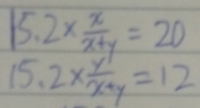ratio confusion

JeffM

Elite Member
What is UNKNOWN is the ratio between X and Y so assign it a letter. Make sense?

[MATH]r = \dfrac{X}{Y}.[/MATH]
Now if we suppose we use 1 liter of Y, how many liters of X do we use? Obviously 1 * r is r. So many liters of the mix do we make? Just as obviously 1 + r.

Now we can think about costs.

[MATH]1 * 12 + r * 20 = (1 + r) * 15.2.[/MATH]
Now what?

Jomo

Elite Member
Sometimes you have to see your mistakes.

How can 15.2x/(x+y) equal both 12 and 20? This can't be!

Last edited:

JeffM

Elite Member
Sometimes you have to see your mistake.

How can 15.2x/(x+y) equal both 12 and 20? This can't be!
@Jomo But psychologically, this is the hardest thing to do. You start down a wrong path because you see no other. And correcting that initial defect in vision just never occurs to you. I have spent days of serious effort trying to prove things that initially seemed to me to be necessarily true but were in fact false.

That is why hints can be helpful: “Oh my, I overlooked that possibility.” We are killed by our sins of omission rather than those of commission.

PS My last comment may be bad theology and bad ethics, but it seems to be true in practice.

•Subhotosh Khan and Jomo

lex

Full Member
Make up a litre of the mixture:
let X be the fraction of a litre of Fruit Juice A that we use,
let Y be the fraction of a litre of Fruit Juice B that we use.
So X+Y=1 i.e. $$\displaystyle \boxed{\,Y=1-X\,}$$ **

Putting the two amounts of juice together gives 1 litre of the mixture.
Now, the cost of the litre of the mixture is:
20X + 12Y = 15.2
Using ** above, $$\displaystyle \hspace2ex$$ 20X + 12(1-X) = 15.2
8X + 12 = 15.2
\displaystyle \begin{align*} \text{X}&= \tfrac{3.2}{8}\\ &= 0.4\\ \end{align*}
So the ratio is 0.4 : 0.6 or 4 : 6 or 2 : 3

Sometimes you have to see your mistakes.

How can 15.2x/(x+y) equal both 12 and 20? This can't be!•Otis, Jomo and jonah2.0

Subhotosh Khan

Super Moderator
Staff member
Make up a litre of the mixture:
let X be the fraction of a litre of Fruit Juice A that we use,
let Y be the fraction of a litre of Fruit Juice B that we use.
So X+Y=1 i.e. [MATH]\boxed{\,Y=1-X\,}[/MATH] **

Putting the two amounts of juice together gives 1 litre of the mixture.
Now, the cost of the litre of the mixture is:
20X + 12Y = 15.2
Using ** above, [MATH]\hspace2ex[/MATH] 20X + 12(1-X) = 15.2
8X + 12 = 15.2
[MATH]\begin{align*} \text{X}&= \tfrac{3.2}{8}\\ &= 0.4\\ \end{align*}[/MATH]So the ratio is 0.4 : 0.6 or 4 : 6 or 2 : 3

View attachment 27978
I think it is just a case of "bad" handwriting. I suppose it should be

$$\displaystyle \frac{15.2 * x}{x + y} \ = 20 \ \$$ and

$$\displaystyle \frac{15.2 * y}{x + y} \ = 12$$

•Jomo